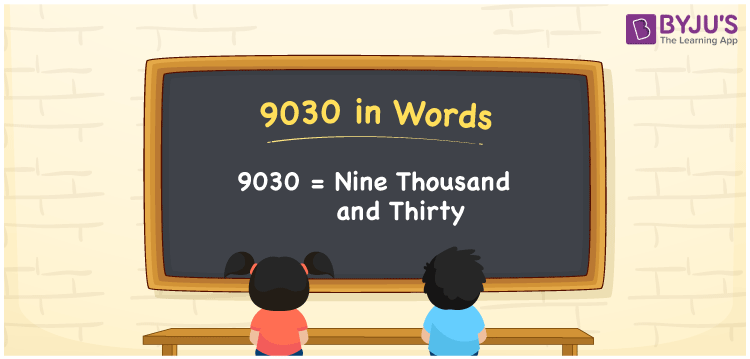# 9030 in Words

9030 in words is written as Nine thousand thirty. In both the International System of Numerals and the Indian System of Numerals, 9030 is written as Nine thousand thirty. The number 9030 is a Cardinal Number as it represents some quantity. For example, “the dress costs 9030 rupees”.

 9030 in Words Nine thousand thirty Nine thousand thirty in Number 9030

## 9030 in English Words

9030 in English words is read as “Nine thousand thirty.”## How to Write 9030 in Words?

To write 9030 in words, we shall use the place value chart. In the place value chart, put 9 in the thousands, 0 in the hundreds, 3 in the tens, and 0 in the ones, respectively. Let us make a place value chart to write the number 9030 in words.

 Thousands Hundreds Tens Ones 9 0 3 0

Thus, we can write the expanded form as

9 × Thousand + 0 × Hundred + 3 × Ten + 0 × One

= 9 × 1000 + 0 × 100 + 3 × 10 + 0 × 1

= 9000 + 0 + 30 + 0

= 9030

= Nine thousand thirty.

9030 is a natural number which is the successor of 9029 and the predecessor of 9031.

9030 in words – Nine thousand thirty

• Is 9030 an odd number? – No
• Is 9030 an even number? – Yes
• Is 9030 a perfect square number? – No
• Is 9030 a perfect cube number? – No
• Is 9030 a prime number? – No
• Is 9030 a composite number? – Yes

## Frequently Asked Questions on 9030 in Words

Q1

### How to write 9030 in words?

9030 in words is written as Nine thousand thirty.
Q2

### How to write 9030 in words in the International and Indian System of Numerals?

In both, the system of numerals, 9030 in words, is written as Nine thousand thirty.
Q3

### How to write 9030 in a place value chart?

In the place value chart, write 9 in the thousands, 0 in the hundreds, 3 in the tens, and 0 in the ones, respectively.